* You are viewing the archive for the ‘@ Formula for Base 10/9 – Pre/Post Octagon – Being/Matter Constants’ Category

## @ Formula for Base 10/9-Pre/Post Octagon-Being/Matter Constants

Derived in this Posting are the Mappings between I-Ching Logic and Base-9 / Base-10 numerical series, which leads to the Topology of I-Ching. Derived also are the Being-Matter Constants, and their connections to each other; namely the author, as the Observer, participate in the (Vacuum) computer in deriving the formulas for these constants. The next 15 Postings are the final (Matured) summary/conclusions of the Matter-Being Paradigm.

Thus, before we begin the next 15 Post-summary, let us again review the following set of understandings on deriving or formulating this Participatory Universe: (1) All formulates are taken from One of Many [Observer—Observed—Reference Frame] Perspectives. (2) No perspective is in a state of truth—accurate—complete. (3) All perspectives are relative to the observer’s understanding / state of interest (at the point of observation) with respect to that particular NOW-state (or many repeat-NOW-states) of mind-brain interaction. (4) All perspectives are depended on the mutual interacting of the NOW-logic between the maturities of the Observer-Observed. Therefore, everyone of the Posting has a slightly different formula of this Observer—Observed—Participatory Universe. In fact, the latest Postings are most matured —-> Measured by the LOVE of GOD.

The following diagrams are formula for Base-9, Base-10, Being-Matter Constants

BASE-9 FORMULA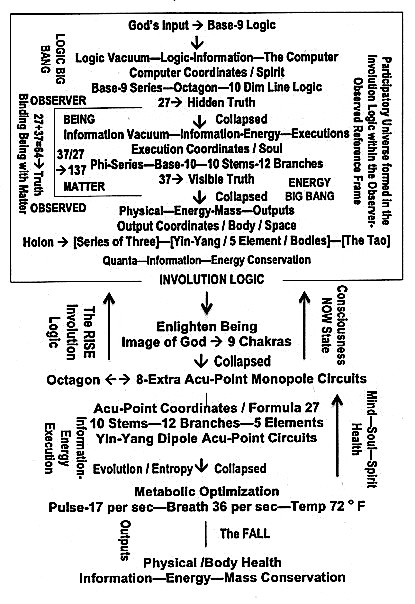The Holon Series inside Base-9, the Logic Vacuum: Love of God —-> Operated by the Power of Series 9, Enlighten Being —-> Operated by the Power of Series 8, while Series 1 represents the Observer. The Three formed the Tao, the Holon in Creating the Participatory Universe. [Series 9 —-> The SPIRIT of the Participatory Universe, Series 8 —-> The Soul of the Participatory Universe, Series 1 —-> The Mind / Brain (Being in Matter, Binding Being with Matter) of the Participatory Universe]

9 —-> GOD’S number, 8 —-> HUMANITY number, 1 —-> The OBSERVER

BEING-MATTER CONSTANTS, DIAGRAM 1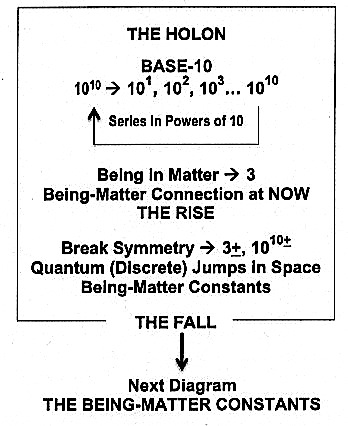DIAGRAM 2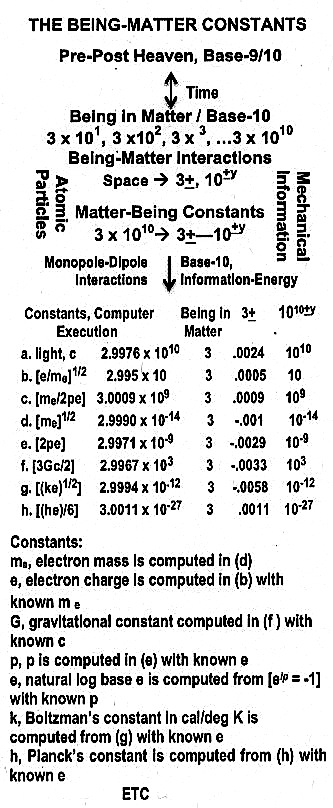DERIVING BASE-9 / BASE-10 AT NOW

Gnomonic Expansion-Contraction-Spiral in Base-9/10: We shall start with the Pre-Heaven Octagon. As shown, there are a total of Eight radial lines. The radial aliments of the number 9 can be arranged in the following way.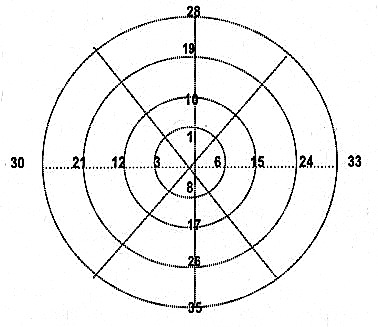As an example, the following pairs of numbers (3, 6), (12, 15), (21, 24), (30, 33) are used to demonstrate the computation. We have: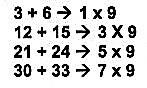The same calculation can be applied for the other 7seven radial lines. There are a total of eight radial lines as shown in the above Diagram.

As for the Post Heaven Octagon, all the numbers, both rational and irrational, are involved in Base 10 for number 10. But these numbers can all be generated with the Holon of 1-2-3. This generation is shown below.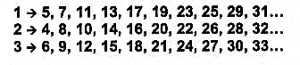Notice, like base-9, these three series can be illustrated on three separate concentrate circles. However, let us combine these three circles into one.

Displayed in three concentric circles, half of the numbers are odd, and embedded in the sum of these odd numbers is the multiplier number 300, or the number 3, which is the constant of light. This result is shown in the following computation. Counting 25 numbers on each circle, and if where the point of intersection between the two circles which is counted twice as a starting and final number, we have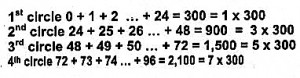Putting these concentric circles into one, we have the following Diagram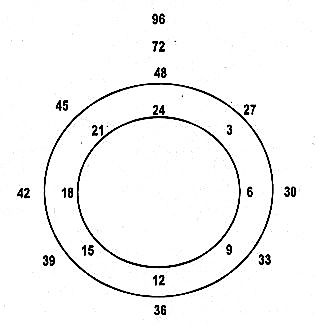Thus, by multiplying the above sequence of odd numbers, i.e., 1, 3, 5, 7… the basic multiplier is 300. Multiplying the above sequence of odd numbers, i.e., 1, 3, 5, 7… and within this odd number sequence is the prime number set.

If we take the sum of these concentric circles, 10 at a time, we would have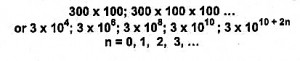Putting the two diagrams together, we have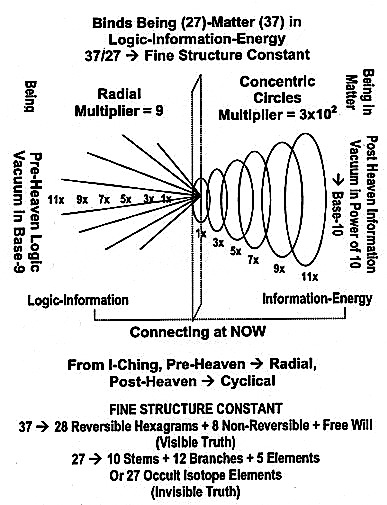To complete the Holon, we need Series for #12 (Series of 6(s)). The above Diagram on Pre-Heaven (Logic Big Bang) and Post-Heaven (Energy Big Bang), which has to do with the Now connections between the Logic Vacuum (Being) and the Information Vacuum (Being in Matter). This has to do with the discrete computer execution in binding Being with Matter; namely the component in-- binging with the two in --and--. The third component has to do with the human as the Left-Right Brain Observer.

This Holon is shown below

Pre-Post Heaven <——> Non-Local / Local Involution Logic

Logic <—— Big Bangs —–> Energy

HUMAN OBSERVER <——> Discrete Changes in Evolution-Entropy

THE TOPOLOGY OF I-CHING: PRE-POST OCTAGON<——> HYPOBOLIC MANIFOLD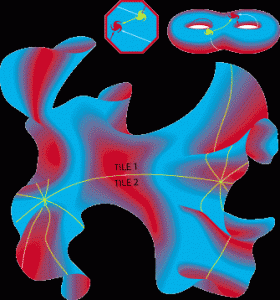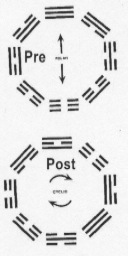LOGIC-ENERGY <——> HYPOBOLIC MANIFOLD

From topology, a finite or infinite hyperbolic object can be formed by an octagon whose opposite sides are connected (in this case by yin-yang logic), so that anything crossing one edge re-enters from the opposite edge, see diagram above. As shown also is how the Pre (Logic-Information Big Bang) and Post (Information-Energy Big Bang) Octagons forming an Involution-Evolution Tetraktys that connects the octagon through the trigrams. Notice that the observers who live on the surface would see an infinite octagonal grid of galaxies, even if space is finite. Such a grid can be drawn only on a hyperbolic manifold – a strange floppy surface where every point has the geometry of a saddle.

Notice, the popular notions of In-Out Vortexes serve as a Theory of Everything are not completely correct. These In-Out Vortexes are formed within the Involution-Evolution Tetraktys, which involves the Platonic Solids.

A HYPOBOLIC UNIVERSE, SEE

### Shape of the Universe – Wikipedia, the free encyclopedia

en.wikipedia.org/wiki/Shape_of_the_Universe‎, Jump to Hyperbolic universe – . A hyperbolic universe is described by hyperbolic geometry, and can be thought of locally as a three-dimensional … ‎Doughnut theory of the universe – ‎Friedmann–Lemaître – ‎Zero-energy universe

AND

### Hyperbolic Universe: Philosophy Forums

forums.philosophyforums.com › … › Hyperbolic Universe‎, 10 posts – 5 authors, Posted 03/25/09 – 7:04 AM: Subject: Hyperbolic Universe This topic came to me whilst posting a reply to a thread about ‘the edge of the Universe’. I want to …

ALSO, I-Ching Logic <——> Topological Strings

### Topological string theory – Wikipedia, the free encyclopedia

en.wikipedia.org/wiki/Topological_string_theory,In theoretical physics,
topological string theory is a simplified version of string theory. The
operators in topological string theory represent the algebra of …
Admissible spacetimes – ‎Objects – ‎Observables – ‎Dualities

We have, therefore, connected the Ancient through I-Ching Logic to Modern and Post Modern through Topology of Strings, and thus connects the Ancient Universe of the Right Brain to the Modern Universe of the Left Brain.